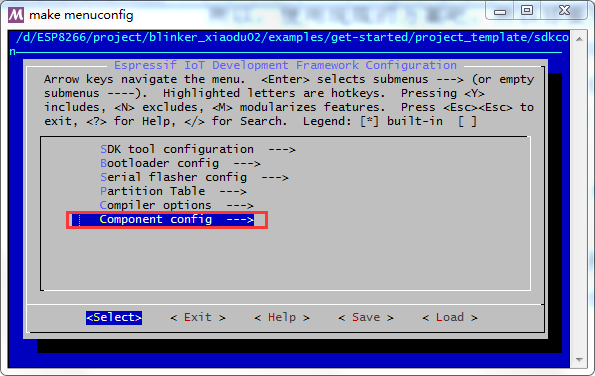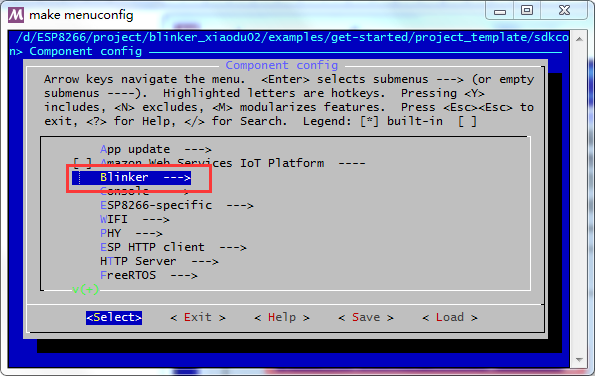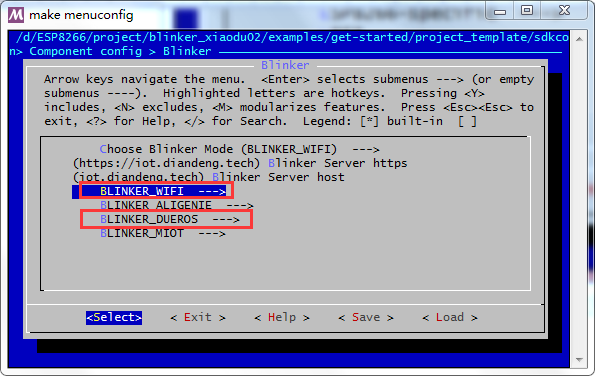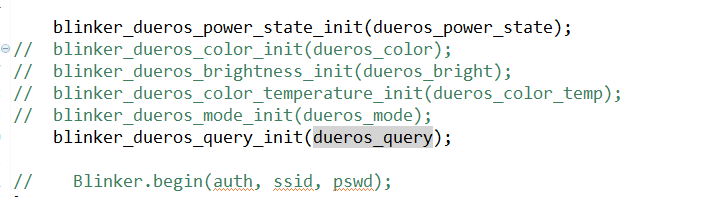wifi的账号、密码，已经模块密钥都在这里填写，程序里就不用再写了。``````void dueros_power_state(const char *state)
{
static int state_flag = 0;
if(state_flag == 0)
{
//GPIO12拉高，GPIO13拉低
gpio_output_set(BIT12, BIT13, BIT12|BIT13, 0);
state_flag = 1;
}
else
{
gpio_output_set(BIT13, BIT12, BIT12|BIT13, 0);
state_flag = 0;
}
BLINKER_LOG(TAG, "need set power state: %s", state);
.    power_state = state,
};
if (strcmp("True", state) == 0) wsState = 1;
else wsState = 0;
}
``````

GPIO操作的那部分是我加的，看到这里该懂的就懂了。说白了就是，收到语音控制开关状态时，执行该函数。

1.牛逼！网上很多都是接入小爱同学，直接用官方的例程，连改都懒得改。你这里直接移植到了小度下面！

回复
•看的挺仔细

回复
•esp8266

回复
•不是这里

回复
2.•